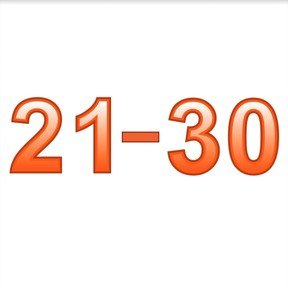Know numbers to 30

# Know numbers to 30

Know numbers to 308,000 schools use Gynzy92,000 teachers use Gynzy1,600,000 students use Gynzy

## General

Students learn to recognize numbers 21 to 30 from their spoken form and can write down the numbers. Students can say the numbers, write the numbers, and write the numbers in words.

1.NBT.A.1

## Relevance

Dsicuss with students that nubmers are all around us and it is important to recognize them. An example is if they want to read the temperature on a thermometer.

## Introduction

Students practice recognizing numbers 11 to 20. The teacher points at a ball and the student says the number.

## Development

The teacher points at the numbers 21-30 and says their names aloud. Ask students to practice saying the number aloud, repeating you. Next the numbers are in random order. Point at a number and ask the students to say the number aloud. Explain how the number must be written. Next the students practice writing the numbers in pairs. The first student selects a key with a number and says the number aloud. The second student writes the number without looking at the interactive whiteboard. Take turns.

Check that students have understood by asking the following questions:
Where might you run into these numbers?
Can you give an example? (street signs, on a ruler, taking a number at the store)
Which number do you see? Teacher can choose to write a few numbers on the interactive whiteboard and ask students to name them.

## Guided Practice

Students first practice by linking a spoken number to a visual number, and then by linking a visual number to a spoken number.

## Closing

Discuss with students why it is useful to recognize numbers to 30. Numbers are everywhere. An example is if you want to read a temperature on a thermometer. Check that students have understood by naming a few numbers between 21-30 and asking students to write them down. Check that students write the numbers correctly and not in reverse order.

## Teaching Tip

Students who have difficulty can practice saying and writing numbers to 20. The students who are ready for a challenge can practice saying and writing numbers to 50.

### The online teaching platform for interactive whiteboards and displays in schools

• Save time building lessons

• Manage the classroom more efficiently

• Increase student engagement